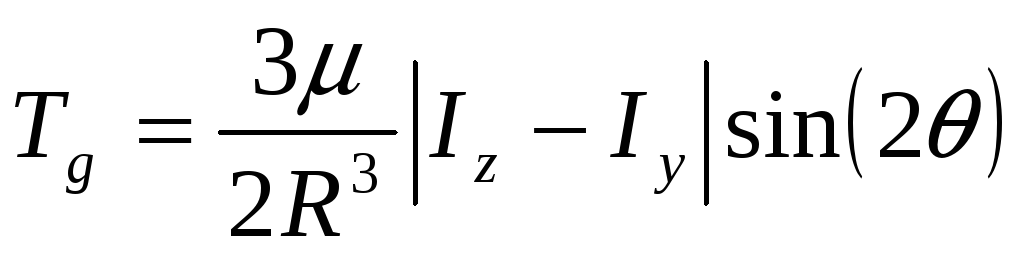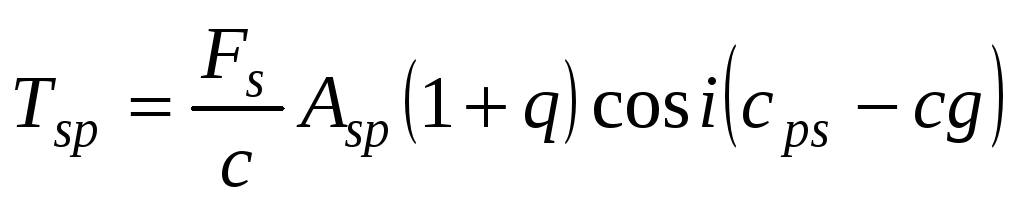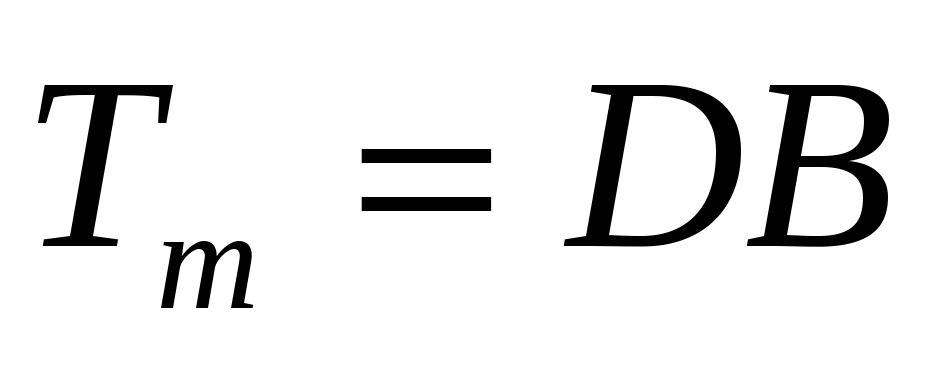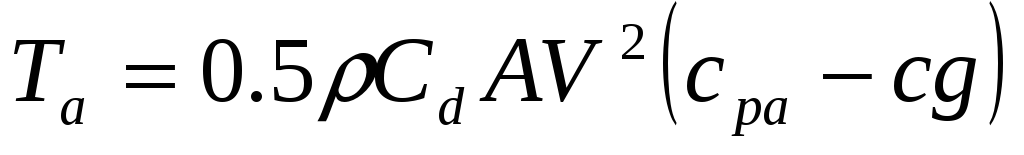# Micromaps host Satellite Design Proposal

 Page 7/11 Date 23.04.2018 Size 1.32 Mb. #46105

## 2.2.5 Component layout

The VASCAT design varies from the HokieSat design by volume due to added components. The MAPS instrument is 0.007 m3 and occupies as much space inside the new satellite. Other added components include the communications hardware required to use the AMSAT frequency and the addition of a camera for high quality photos. The VASCAT grows with the added components.

In addition to increased volume constraints, mounting constraints accompany the instrument. The instrument’s lens must be nadir pointing at all times, and its feet are located on an adjacent side to its lens. Therefore, the MAPS instrument must be mounted to a vertical interface and look through a hole in the bottom panel. Isogrid modification or addition of a vertical shelf is considered as component mounting solutions in the following trade study. The following lists the parameters used in the trade study:

1. Mass: This represents the mass added to the satellite due to the configuration change. This parameter is weighted on a scale of 1 to 10, with 10 representing negligible added mass

2. Manufacturing cost: This represents how much the production of this configuration addition costs. This parameter is weighted on a scale of 1 to 10, with 10 being no added cost

3. Ease in mounting: This represents a measure of how trivial attachment of components to the structure will be. For example, threaded inserts are complicated while fasteners are fairly simple. This parameter is weighted on a scale of 1 to 10, with 10 being the easiest to mount.

Weights are assigned to each parameter to signify its level of importance. Table 6 shows the results of this trade study. The option with the highest weight score is desired. Modified isogrid is chosen for component mounting.

Table 6: Trade study of component mounting techniques

 Selection parameter Weight Modified isogrid Center component shelf Rating Weighted score Rating Weighted score Mass 20 9 180 5 100 Cost 40 5 200 7 280 Simplicity 10 7 70 1 10 Totals 450 390

The ADCS stabilizes and maintains the attitude of the VASCAT. An ADCS consists of sensors and actuators. The sensor measurements are used to calculate the attitude of the satellite. The calculated attitude is compared to a desired attitude and the proper inputs are given to the attitude actuators. The actuators apply a torque to the satellite to reach the desired attitude.

## 2.3.1 Attitude control architecture

The VASCAT uses a single wheel momentum-bias attitude control system. The momentum wheel’s spin axis is aligned normal to the orbital plane. It controls the attitude about the satellite’s pitch axis and stabilizes the attitude about the yaw and roll axes. Three magnetic torque bars (MTB) are used to perform momentum-dumping maneuvers when the momentum wheel reaches its momentum storage limit. The MTBs are arranged in a triad to create a magnetic torque in any direction, except the direction of the magnetic field.

Attitude knowledge is required to be accurate within 0.5 degrees by the MAPS instrument.19 Attitude determination is performed using two Earth sensors, two sun sensors, three rate gyros, and a magnetometer. The attitude requirements imposed by the MAPS instrument are summarized in Table 7.

Table 7: MicroMAPS imposed attitude requirements19

 Requirement Value Pointing Direction Nadir pointing Control accuracy (3)  2.5 Knowledge accuracy (3)  0.5

## 2.3.2 Attitude control modes

The VASCAT uses three modes of attitude control. The satellite operates in normal mode during the majority of its mission. A momentum-dump mode is entered whenever the momentum wheel reaches its upper limit of momentum storage. The satellite’s computer initiates safe mode should it experience system level error.

2.3.2.1 Normal

The ADCS of the satellite operates in normal mode during the majority of its mission. The attitude of the satellite is determined every ten seconds and transmitted to a ground station. All available sensor data is used to determine attitude to ensure that the attitude knowledge is accurate to 0.5 degrees. Attitude knowledge is compared with the desired attitude and a control torque is applied using the momentum wheel or magnetic torque bars accordingly. This ensures that the pointing error is kept below 2.5 degrees.

2.3.2.2 Momentum-dump

The satellite uses a momentum wheel for attitude control and stabilization. Over time secular attitude disturbances build up momentum in the wheel. When the build up of momentum reaches the storage limit of the momentum wheel, a momentum-dumping maneuver is performed. The three MTBs are used to apply an external torque to the satellite so that the momentum wheel applies an equal and opposite torque to lower its spin rate. The attitude determination system performs as it does in normal mode, but the magnetometer readings are not used for attitude determination while the MTBs are active.

2.3.2.3 Safe

The satellite enters safe mode if an error occurs during operation. The satellite’s computer determines the entry and exit of this mode. During this mode the ADCS is passive. The momentum stored in the momentum wheel and the gravity-gradient stabilize the satellite.

## 2.3.3 Disturbance torques

The VASCAT encounters disturbance torques on orbit. It is subjected to solar pressure, gravity-gradient, atmospheric drag, and magnetic torques. A significant torque is due to the gravity-gradient. The force of gravity varies with an object’s distance from the center of the Earth. The lower sections of the satellite feel a greater gravitational force compared to the upper sections. The result is a torque exerted on the satellite if it is not directly nadir pointing.2-920

The moments of inertia, Iz and Iy, of the satellite as well as its radius, R, and the angle off nadir, , determine the magnitude of the torque.

Solar pressure is exerted on the satellite by photons, emitted from the Sun, that strike the satellite. The force is exerted at the center of solar pressure of the satellite. If the center of solar pressure and center of gravity do not coincide then a torque is created.2-1020

The surface area exposed to the sun, Asp, the center of solar pressure, cps, the center of gravity, cg, and the reflectance factor, q, are the satellite properties that determine the torque. The solar constant, Fs, and the speed of light, c, are the constants that influence the magnitude of the torque.

The electronics on-board the VASCAT induce a magnetic dipole during operation. The magnetic dipole interacts with the geomagnetic field to produce a torque. The maximum torque occurs when the magnetic dipole direction is perpendicular to the geomagnetic field.2-1120

The worst case magnitude of the torque is calculated using Equation 2-11, where D is the strength of the magnetic dipole and B is the magnitude of the local geomagnetic field.

Although the atmosphere in Low-Earth Orbit (LEO) is sparse, the high velocity of a satellite creates some drag. The drag force acts at the center of pressure of the satellite. If the center of pressure does not coincide with the center of gravity, the drag creates a torque.2-1220

The torque is equal to the drag force times the length of the moment arm, or the distance between the center of atmospheric pressure, cpa, and the cg. Equation 2-12 is used to calculate the magnitude of the torque, where is the atmospheric density, Cd is the drag coefficient, A is the projected area, and V is the velocity of the satellite.

Preliminary estimates of the structural properties and orbital parameters of the satellite are used to estimate these torques. Table 8 summarizes the structural properties used in the calculations.

Table 8: Estimated structural properties of the VASCAT

 Description Value Ix 4.4 kg/m2 Iy 4.4 kg/m2 Iz 1.9 kg/m2 As 0.18 m2 cg 0.10 m c.p. 0.17 m Cd 2.5

The estimated orbital parameters used in the calculations for disturbance torques are summarized in Table 9.

Table 9: Estimated orbital properties of the VASCAT

 Description Value R 6778 km  2.72  10-12 kg/m2

The disturbance torques are estimated using equations 2-9 through 2-12 above. A simple Matlab code is used to perform the calculations, and is presented in Appendix D. Table 10 summarizes the results of the calculations.

Table 10: Estimated disturbance torques

 Disturbance Torque (N-m) Gravity gradient 4.8 × 10-6 Solar pressure 8.5 × 10-8 Magnetic 5.1 × 10-5 Atmospheric drag 2.3 × 10-12

The magnitude of the total disturbance torque is estimated to be 56 N-m. The majority of the disturbance torque is due to the magnetic torque created by the interaction of the satellite’s electronics with the geomagnetic field. A magnetic dipole of 1 A-m2 was assumed for the magnetic disturbance torque calculation. The second largest component is due to the gravity-gradient. The VASCAT is nadir pointing. In this orientation the torque due to the gravity-gradient is zero and acts as a restoring torque in case of deviation from nadir.